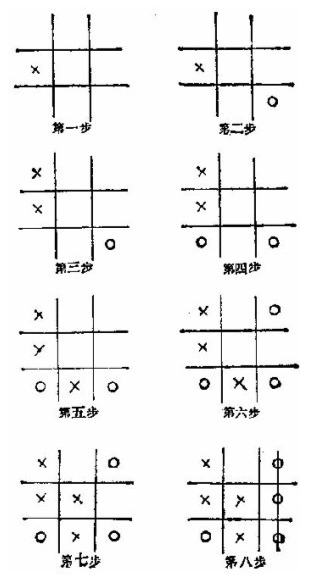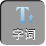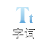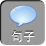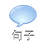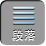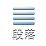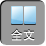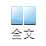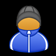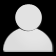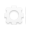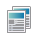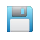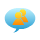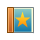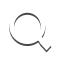-AA+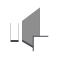1+ 5+ 9= 15

1+ 6+ 8= 15

2+ 4+ 9= 15

2+ 5+ 8= 15

2+ 6+ 7= 15

3+ 4+ 8= 15

3+ 5+ 7= 15

4+ 5+ 6= 15

2 9 4

7 5 3

6 1 8